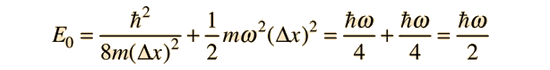# Quantum Harmonic Oscillator: Energy Minimum from Uncertainty Principle

The ground state energy for the quantum harmonic oscillator can be shown to be the minimum energy allowed by the uncertainty principle.

The energy of the quantum harmonic oscillator must be at leastTaking the lower limit from the uncertainty principleThen the energy expressed in terms of the position uncertainty can be writtenMinimizing this energy by taking the derivative with respect to the position uncertainty and setting it equal to zero givesSolving for the position uncertainty givesSubstituting gives the minimum value of energy allowed.This is a very significant physical result because it tells us that the energy of a system described by a harmonic oscillator potential cannot have zero energy. Physical systems such as atoms in a solid lattice or in polyatomic molecules in a gas cannot have zero energy even at absolute zero temperature. The energy of the ground vibrational state is often referred to as "zero point vibration". The zero point energy is sufficient to prevent liquid helium-4 from freezing at atmospheric pressure, no matter how low the temperature.

Index

Schrodinger equation concepts

Reference
Rohlf
Sec 7-5

 HyperPhysics***** Quantum Physics R Nave
Go Back